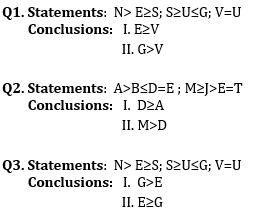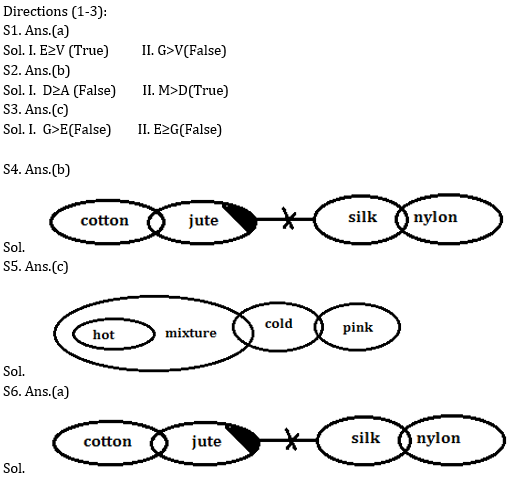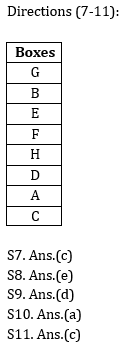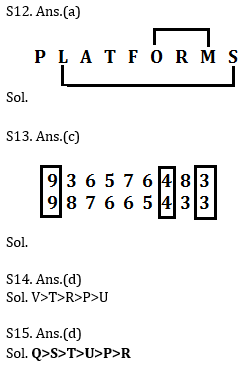# Reasoning Ability Quiz for IBPS Clerk Prelims Exams- 8th December

Directions (1-3): In these questions, relationship between different elements is shown in the statements. The statements are followed by two conclusions. Give the answer:
(a) If only conclusion I is true
(b) If only conclusion II is true
(c) If either conclusion I or II is true
(d) If neither conclusion I nor II is true
(e) If both conclusions I and II are trueDirections (4-6): In each of the questions below are given some statements followed by two conclusions. You have to take the given statements to be true even if they seem to be at variance with commonly known facts. Read all the conclusions and then decide which of the given conclusions logically follows from the given statements disregarding commonly known facts.

Q4. Statements: Only a few cotton is jute. Some jute is not silk. Some silk is nylon.
Conclusions: I. All jute being nylon is a possibility.
II. Some cotton is not jute.
(a) If only conclusion II follows.
(b) If both conclusions I and II follow.
(c) If either conclusion I or II follows.
(d) If only conclusion I follows.
(e) If neither conclusion I nor II follows.

Q5. Statements: Some mixture is cold. Only a few cold is pink. Only mixture is hot.
Conclusions: I. All mixture being pink is a possibility.
II. Some mixture can never be cold.
(a) If either conclusion I or II follows.
(b) If both conclusions I and II follow.
(c) If only conclusion II follows.
(d) If only conclusion I follows.
(e) If neither conclusion I nor II follows.

Q6. Statements: Only a few cotton is jute. Some jute is not silk. Some silk is nylon.
Conclusions: I. Some silk is not nylon.
II. All silk is nylon.
(a) If either conclusion I or II follows.
(b) If both conclusions I and II follow.
(c) If only conclusion II follows.
(d) If only conclusion I follows.
(e) If neither conclusion I nor II follows.

Directions (7-11): Study the following information carefully and answer the given questions.
There are eight boxes i.e. A, B, C, D, E, F, G and H which are kept one above the other but not necessarily in the same order. Three boxes are placed between box F and box C. Box F is placed above box C. Box B is placed at second position from the top. Box E is placed just above the box F. Only one box is placed between box H and box A. Box D is placed just above box A.

Q7. Which of the following boxes is placed at the bottommost position?
(a)G
(b)E
(c)C
(d)A
(e)None of these

Q8. How many boxes are placed between box D and box G?
(a)Two
(b)One
(c) Three
(d)None
(e) More than three

Q9. Which of the following statements is true about box G?
(a) Two boxes are placed above box G
(b) Box A is placed just above box G
(c) Three boxes are placed between box E and Box G
(d) Box G is placed at the topmost position
(e) All are true

Q10. Which of the following box is placed just above the box D?
(a) H
(b) B
(c) G
(d) C
(e)None of these

Q11. What is the position of box A?
(a) Just below box G
(b)At third position from the top
(c) Placed below box F
(d) None of these
(e) At fourth position from the bottom

Q12. How many pairs of letters are there in the word “PLATFORMS” which has as many letters between them as we have in the English alphabetical series (from both forward and backward direction)?
(a) Two
(b) One
(c) Three
(d) None
(e) More than three

Q13. How many such numerals are there in the number ‘936576483’ which will remain at the same position when they are arranged in ascending order from right to left?
(a) One
(b) Two
(c) Three
(d) more than three
(e) None

Q14. P, U, V, R and T have different heights. T is just taller than R but just shorter than V.P is taller than U.P is not the tallest person then who among the following is the second tallest person?
(a) P
(b) V
(c) U
(d)T
(e) R

Q15. Six boxes i.e. P, Q, R, S, T and U are arranged according to their weight in descending order. Book P is heavier than only book R. Book T is heavier than book U.  Book S is heavier than book T but not the heaviest among all. Which among the following is the third lightest book?
(a) Q
(b) S
(c) T
(d) U
(e) None of these

Practice More Questions of Reasoning for Competitive Exams:

##### Prelims Quiz Study Plan for SBI & IBPS Exams 2020

SolutionsPractice with Crash Course and Online Test Series for IBPS Clerk Prelims: